Visitors Online: 77 | Friday 24th May 2019

CBSE Guess > Papers > Question Papers > Class XII > 2004 > Chemistry > Delhi Set-I

CHEMISTRY (Set I—Delhi)

Q. 1. Name a substance which on addition to AgCl causes vacation vacancy in it. 1

Q. 2. Mention a large scale use of the phenomenon called ‘reverse osmosis’. 1

Q. 3. Give an example of a pseudo first order reaction. 1

Q. 4. Write the JUPAC name of the following: (CH3)2 C=CHCOCH3

Q. 5. Mention two properties of acetonitrile b of which it acts as a good solvent. 1

Q. 6. Draw a diagram showing the formation of bonding and antibonding molecular orbitals by the LCAO in homonuclear hydrogen molecule. 2

Q. 7. Taking a specific example show that AS total is a criterion for spontaneity of a change. 2

Q. 8. Write the cell reactions which occur in lead storage battery (i) when the battery is in use (ii) when the battery is on charging. 2

Q. 9. Give chemical reaction in support of each of the following statements: 2
(i) The +1 oxidation state gets stabilised progressively from Ga to TI in Group
(ii) All the bonds PCl5 are not equivalent.

Q. 10. Explain the following terms: 2
(a) Asymmetric molecule
(b) R and S notations

Q. 11. Write the names of the reagents and equations in the conversion of 2
(a) phenol to salicyl aldehyde
(b) anisole to p-methoxyacetophenone

Q. 12. Write the modes of free radical polymerization of an alkene.

Or

Differentiate between addition and condensation polymers based on mode of Polymerization. Give one example of each type. 2

Q.13. What information is given by (i) Radial probability density, R2 and (ii) Radial probability function,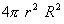in hydrogen atom? How do they vary with r for 1s orbital of hydrogen atom? Show diagrammatically. 3

Q. 14. Calculate the density of silver which crystallizes in the face-centred cubic structure. The distance between the nearest silver atoms in this structure is 287 pm.3
(Molar mass of Ag = 107.87 g mol-1, NA 6.02 x 1023 mol-1)

Q. 15. Two elements A and B form compounds having molecular formulae AB2 and AB4. dissolved in 2O g of benzene, 1 g of AB2 lower the freezing point by 2.3 K, whereas 1 g of AB4 lowers it by 1.3 K. The molar depression constant for benzene is 5.1 K kg mor-1. Calculate the atomic masses of A and B. 3

Q. 16. Calculate the standard gibbs energy change for the formation of propane at 298 k.

Q. 17. Conductivity of 0.00241 M acetic acid is 7.896 x 10-5 S cm-1. Calculate its mlaor conductivity. If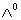for acetic acid is 390.5 S cm2 mol-1, what is its disso-ciation constant? 3

Q. 18. A reaction is first order in A and second order in B.
(a) Write differential rate equation.
(b) How is the rate affected if the concentration of B is tripled?
(c) How is the rate affected when the concentrations of both A and B are doubled?
What is the significance of rate constant In the rate expression? 3

Q. 19. How are the colloids classified on the basis of the nature of interaction between dispersed phase and dispersion medium? Describe an important characteristic of each class. Which of these sole need stabilising agents for preservation? 3

Or

What are detergents? Give their scheme classification. Why are the detergen- ts preferred over soaps?

Q. 20. Draw a figure to show splitting of degenerate d orbitals in an octahedral crystal field. How does the magnitude of decide the actual configuration old orbitals in a complex entity? 3

Q. 21. Write the nuclear reactions for the following radioactive decay: 3
(i)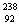U undergoes an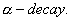(ii)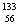Ba undergoes K-capture.

(iii)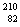Pb undergoes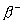- decay.

Q.22. Draw the structure Of a carbonyl group and indicate clearly (i) the hybridized state of carbon, (ii) the a and bonds present and (iii) the electrophilic and nucleophilic centres in it. 3

Q. 23. (a) Identify A and B in the following: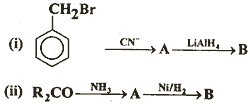(b) Explain why the aromatic amines are less basic than ammonia and alipha-tic amines. 3

Q. 24. On the basis of application, how are dyes classified? Describe essential feat-ures of any two of them. 3

Q. 25. (i) Assign appropriate reason for each of observations:
(a) Anhydrous ALCI3 is used as a catalyst.
(b) Phosphinic acid behaves as a monoprotic acid.
(c) SF6 is not easily hydrolysed whereas SF4 is readily hydrolysed.
(d) No form of elemental silicon is comparable to graphite.

(ii) Draw the structure of XeOF4 or BrF3. 5

Q. 26. (i) How would you account for the following:
(a) The transition elements exhibit high enthalpies of atomization.
(b) The 4d and 5d series of the transition metals have more frequent met- al metal bonding in their compounds than do the 3d metals.
(c) There is a greater range of oxidation states among the actinoids than that in the lanthanoids.

(ii). Write the complete chemical equation for each of the following:
(a) An alkaline solution of KMnO4 reacts with an iodide.
(b) An excess of SnCI2 solution - added ton solution of mercury (II) chloride. 5

Q. 27. Name the products obtained on complete hydrolysis of DNA. Enumerate the structural differences between DNA and RNA. In what way is a nucleotide dif-ferent from a nucleoside? Illustrate with examples. 5

Or

Define and classify vitamins. Name the diseases caused due to lack of any three of them.

 Chemistry 2004 Question Papers Class XII Delhi Outside Delhi Compartment Delhi Compartment Outside Delhi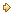Set ISet ISet ISet ISet IISet IISet IISet IISet IIISet III

CBSE 2004 Question Papers Class XII